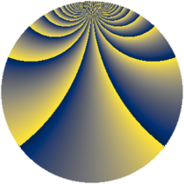# Properties

 Label 1344.4.cwLevel $1344$ Weight $4$ Character orbit 1344.cw Rep. character $\chi_{1344}(5,\cdot)$ Character field $\Q(\zeta_{48})$ Dimension $12224$ Sturm bound $1024$

# Related objects

## Defining parameters

 Level: $$N$$ $$=$$ $$1344 = 2^{6} \cdot 3 \cdot 7$$ Weight: $$k$$ $$=$$ $$4$$ Character orbit: $$[\chi]$$ $$=$$ 1344.cw (of order $$48$$ and degree $$16$$) Character conductor: $$\operatorname{cond}(\chi)$$ $$=$$ $$1344$$ Character field: $$\Q(\zeta_{48})$$ Sturm bound: $$1024$$

## Dimensions

The following table gives the dimensions of various subspaces of $$M_{4}(1344, [\chi])$$.

Total New Old
Modular forms 12352 12352 0
Cusp forms 12224 12224 0
Eisenstein series 128 128 0

## Trace form

 $$12224q - 24q^{3} - 16q^{4} - 32q^{7} - 8q^{9} + O(q^{10})$$ $$12224q - 24q^{3} - 16q^{4} - 32q^{7} - 8q^{9} - 48q^{10} - 24q^{12} - 32q^{15} - 16q^{16} - 8q^{18} - 48q^{19} - 16q^{21} - 64q^{22} - 24q^{24} - 16q^{25} - 32q^{28} + 2312q^{30} - 1792q^{36} - 16q^{37} - 8q^{39} - 48q^{40} + 3144q^{42} - 64q^{43} - 24q^{45} - 16q^{46} - 32q^{49} - 8q^{51} - 9984q^{52} - 24q^{54} - 32q^{57} - 16q^{58} - 8q^{60} - 48q^{61} - 32q^{63} - 12160q^{64} - 24q^{66} - 6544q^{67} - 32q^{70} - 8q^{72} - 48q^{73} - 24q^{75} - 32q^{78} - 16q^{79} - 8q^{81} - 20928q^{82} + 4128q^{84} - 64q^{85} - 24q^{87} - 16q^{88} - 32q^{91} + 424q^{93} - 48q^{94} + 38736q^{96} - 32q^{99} + O(q^{100})$$

## Decomposition of $$S_{4}^{\mathrm{new}}(1344, [\chi])$$ into newform subspaces

The newforms in this space have not yet been added to the LMFDB.